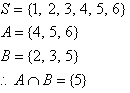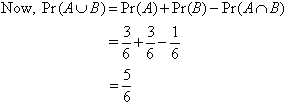Year 10 Interactive Maths - Second Edition

## Mutually Exclusive Events

A and B are said to be mutually exclusive events if they do not overlap. This means that A and B are mutually exclusive events such that if A occurs then B is excluded or if B occurs then A is excluded. That is, A and B cannot occur together.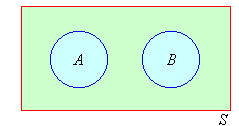###### Note:

Mutually exclusive events have no sample points in common.

Consider the experiment of throwing a die.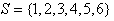Let A be the event that an odd number is obtained and B be the event that an even number is obtained. Then: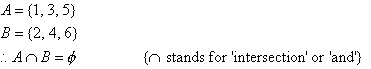That is, A and B have no elements (sample points) in common. Hence A and B are mutually exclusive events, as shown in the following Venn diagram.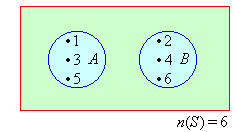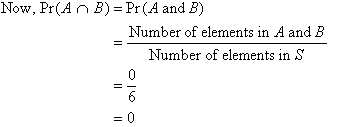## Addition Law of Probabilities

For the example under consideration: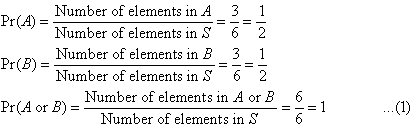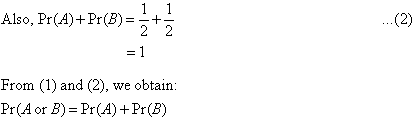###### Note: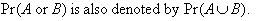###### In general: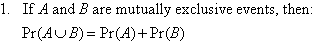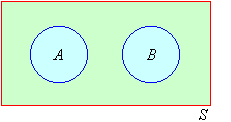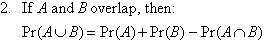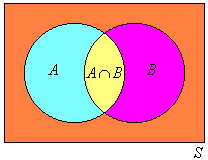Consider the experiment of throwing a die. As usual:Let the events be defined as follows:

A = the event that an even number is obtained; and
B = the event that a prime number is obtained.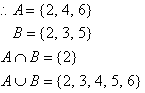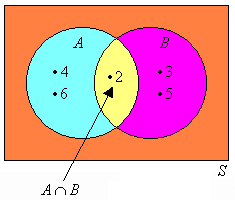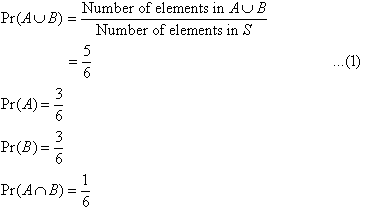We notice that: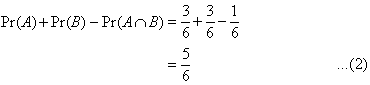From (1) and (2), we obtain: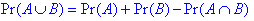This is called the addition law of probabilities.

#### Example 6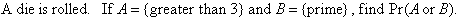##### Solution: